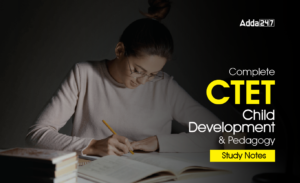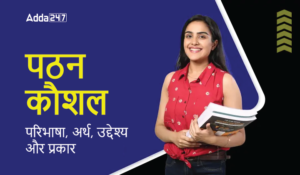# Light (Reflection): Download Science Study Notes FREE PDF for Teaching ExamsIn many teaching exams including  REETUTET DSSSB Exams etc. Science may be an interesting subject with interesting notes and quizzes. In TET Exams, there will be 30 questions i.e. 15 Questions Science content and 15 questions of Science Pedagogy in REETUTET  and other State TET Exams. Science comprising of various branches of studies like chemistry, physical science, and life science.

Get free Study material for REET Exam

## LIGHT (REFLECTION)

• Light is a form of energy, which gives us the power of vision.
• Ray of light: It is a line the direction of movement of light.
• Beam of light: It is bunch of rays of light.
• Parallel beam: All the rays are parallel.

Reflection of Light:

When the light in allowed to fall on highly polished surface, such as mirror, most of the light gets reflected.

Laws of Reflection:

The Angle of incidence is always equal to angle of reflection• The incident ray, reflected ray and the normal to the reflecting surface the point of incidence lie in the same plane.
• Image formed by Plane Mirror (Plane reflecting surface)
• Virtual (Imaginary and) Erect: the image that does not form on screen. Real images can be recorded in the screen.
• Laterally inverted (The left side of object appears on right side of image)
• The size of image is equal to that of object.
• The image formed is as far behind the mirror as the object is in front of it.

#### How To Score 25+ in Science For CTET Exam

Refection of light by spherical Mirrors:

Mirrors, whose reflecting surface are curved inward or outward spherically are called spherical mirror. For example-Spoon the curved surface of shinning spoon can be considered as curved mirror.If it is curved inward Act as concave mirror

If it is curved outward Act as a convex mirror.

Few Basic terms related to Spherical mirror :

• Principal axis: Line joining the pole and centre of curvature of the spherical mirror.
• Pole: the geometrical central point of the reflecting spherical surface. (Aperture) denoted by P.
• Aperture: The width of reflecting spherical surface.
• Centre of curvature: The centre of the hollow glass sphere of which the spherical mirror is a part is called as centre curvature.
• Radius of curvature: The distance between the pole and the centre of curvature. i.e. PC=R or The radius of the hollow sphere of which the mirror is a part
• Focus point: The point on the principal axis, where all parallel rays meet after reflection is called as Principal Focus or Focus. It is denoted by letter ‘F’.
• Focal Length: The distance between the pole and focus point i.e. PF=f

Image Formation by spherical Mirror:

• Ray of light which is parallel to principle axis always pass through focus (meet at focus) or Vice versa.
• A ray of light which passes through centre of curvature it is also known as normal at the point of incidence of spherical mirror will retrace their path after reflection.
• A ray of light falling on pole get reflected at the same angle on the other side of principal axis.

Note: A ray of light passes through centre of curvature of reflecting spherical surface always act as normal at the point of incidence. If we know the normal we can draw angle of incidence and angle of reflection.

#### Check Complete Science Study Now HERE

Image Formation by Concave Mirror:Image formation by Convex Mirror:Uses of Concave Mirror:

1.Used in torches, search light and headlight of vehicle.

2.Used to see large image of face as shaving mirror

3.Used by dentist to see large images of the teeth

4.Large concave mirror used to focus sunlight (heat) in solar furnaces.

Uses of Convex Mirror:

Used as rear-view mirror in vehicles because it gives erect image. It also helps the driver to view large area.

Sign Convention for Reflection by Spherical Mirror:

• The object is always placed to the left side of mirror.
• All distance should be measured from pole (P); parallel to principal axis.

Right of the origin (+x-Axis) are taken positive

Left of the origin (–x-Axis) are taken negative

#### Check Complete EVS Study Now: HERE

Mirror Formula:Few tips to remember sign convention for Spherical mirror

 f u v CONCAVE -ve(real) -ve(real) -ve(real) +ve(virtual) CONVEX +ve +ve +ve

h – Is always +ve

h´ – is +ve for virtual, –ve for Real.

•अलंकार - परिभाष�...
•विशेषण- परिभाष�...
•लेखन कौशल की पर�...
•Complete CTET Child Development and Peda...
•New Education Policy(NEP) PDF - नई �...
•पठन कौशल- परिभा�...